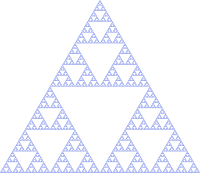The intuition for `FlatMap` and `Monad` we built on day 5 via the tightrope walking example is that a monadic chaining `>>=` can carry context from one operation to the next. A single `None` in an intermediate value banishes the entire chain.

The context monad instances carry along is different. The `State` datatype we saw on day 7 for example automates the explicit passing of the state object with `>>=`.

This is a useful intuition of monads to have in comparison to `Functor`, `Apply`, and `Applicative`, but it doesn’t tell the whole story.Another intuition about monads (technically `FlatMap`) is that they are fractals, like the above Sierpinski triangle. Each part of a fractal is self-similar to the whole shape.

Take `List` for example. A `List` of `List`s can be treated a flat `List`.

``````val xss = List(List(1), List(2, 3), List(4))
// xss: List[List[Int]] = List(List(1), List(2, 3), List(4))

xss.flatten
// res0: List[Int] = List(1, 2, 3, 4)
``````

The `flatten` function embodies the crunching of the `List` data structure. If we think in terms of the type signature, it would be `F[F[A]] => F[A]`.

#### List forms a monad under ++

We can get a better idea of the flattening by reimplementing it using `foldLeft`:

``````xss.foldLeft(List(): List[Int]) { _ ++ _ }
// res1: List[Int] = List(1, 2, 3, 4)
``````

We can say that `List` forms a monad under `++`.

#### Option forms a monad under?

Now let try to figure out under what operation does `Option` form a monad:

``````val o1 = Some(None: Option[Int]): Option[Option[Int]]
// o1: Option[Option[Int]] = Some(value = None)

val o2 = Some(Some(1): Option[Int]): Option[Option[Int]]
// o2: Option[Option[Int]] = Some(value = Some(value = 1))

val o3 = None: Option[Option[Int]]
// o3: Option[Option[Int]] = None
``````

And here’s the `foldLeft`:

``````o1.foldLeft(None: Option[Int]) { (_, _)._2 }
// res2: Option[Int] = None

o2.foldLeft(None: Option[Int]) { (_, _)._2 }
// res3: Option[Int] = Some(value = 1)

o3.foldLeft(None: Option[Int]) { (_, _)._2 }
// res4: Option[Int] = None
``````

It seems like `Option` forms a monad under `(_, _)._2`.

#### State as a fractal

If we come back to the `State` datatype from the point of view of fractals, it becomes clear that a `State` of `State` is also a `State`. This property allows us to create mini-programs like `pop` and `push`, and compose them into a larger `State` using `for` comprehension:

``````def stackManip: State[Stack, Int] = for {
_ <- push(3)
a <- pop
b <- pop
} yield(b)
``````

We also saw similar composition with the `Free` monad.

When you want to find your own monad, keep a lookout for the fractal structure. From there, see if you can implement the `flatten` function `F[F[A]] => F[A]`.